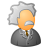# PostGre ST_RASTER Error getValue

318
0
08-24-2017 10:22 AMNew Contributor II

Hi

when i execute

SELECT getValue(raster,1,0,se_coord(-75,4)) r,
FROM [Scheme].[RasterDataSet] WHERE raster_id(raster) = 1;

the GDB say:

NOTICE: ESRI: ST_Coord start
NOTICE: ESRI: ST_Coord end
NOTICE: ESRI: ST_Raster_getValue start
NOTICE: ESRI: prepare SELECT count(*) FROM INFORMATION_SCHEMA.tables WHERE lower(TABLE_CATALOG) = current_database() AND lower(TABLE_SCHEMA) = current_schema() AND lower(TABLE_NAME) = lower(\$1)
NOTICE: ESRI: prepare returned success
NOTICE: ESRI: prepare select srid from sde.sde_raster_columns where rastercolumn_id = \$1
NOTICE: ESRI: prepare returned success
NOTICE: ESRI: prepare select row_nbr, col_nbr, block_data from sde_blk_22 where rasterband_id = \$1 and rrd_factor = \$2 and row_nbr >= \$3 and row_nbr <= \$4 and col_nbr >= \$5 and col_nbr <= \$6

the last select dont have [Scheme] and does not run well

the correct sentence id select row_nbr, col_nbr, block_data from [Scheme].sde_blk_22

but the sentence is controlled by ST_Raster Lib

0 Replies